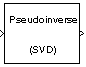# Pseudoinverse

Compute Moore-Penrose pseudoinverse of matrix

## Library

Math Functions / Matrices and Linear Algebra / Matrix Inverses

`dspinverses`

•## Description

The Pseudoinverse block computes the Moore-Penrose pseudoinverse of input matrix A.

```[U,S,V] = svd(A,0) % Equivalent MATLAB code ```

The pseudoinverse of A is the matrix ${A}^{†}$ such that

`${A}^{†}=V{S}^{†}{U}^{\ast }$`

where U and V are orthogonal matrices, and S is a diagonal matrix. The pseudoinverse has the following properties:

• $A{A}^{†}={\left(A{A}^{†}\right)}^{\ast }$

• ${A}^{†}A={\left({A}^{†}A\right)}^{\ast }$

• $A{A}^{†}A=A$

• ${A}^{†}A{A}^{†}={A}^{†}$

## Parameters

Show error status port (E)

Select to enable the E output port, which reports a failure to converge. The possible values you can receive on the port are:

• `0` — The pseudoinverse calculation converges.

• `1` — The pseudoinverse calculation does not converge.

If the pseudoinverse calculation fails to converge, the output at port X is an undefined matrix of the correct size.

Simulate using

Type of simulation to run. You can set this parameter to:

• `Interpreted execution` (default)

Simulate model using the MATLAB®  interpreter. This option shortens startup time. For this block, the simulation speed in this mode is faster than in ```Code generation```.

• `Code generation`

Simulate model using generated C code. The first time you run a simulation, Simulink® generates C code for the block. The C code is reused for subsequent simulations, as long as the model does not change. This option requires additional startup time but the simulation speed increases with subsequent simulations.

## References

Golub, G. H., and C. F. Van Loan. Matrix Computations. 3rd ed. Baltimore, MD: Johns Hopkins University Press, 1996.

## Supported Data Types

PortSupported Data Types

A

• Double-precision floating point

• Single-precision floating point

X

• Double-precision floating point

• Single-precision floating point

E

• Boolean

 Cholesky Inverse DSP System Toolbox LDL Inverse DSP System Toolbox LU Inverse DSP System Toolbox Singular Value Decomposition DSP System Toolbox `inv` MATLAB